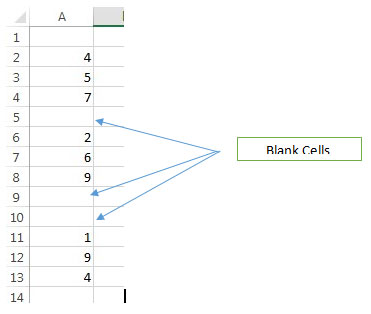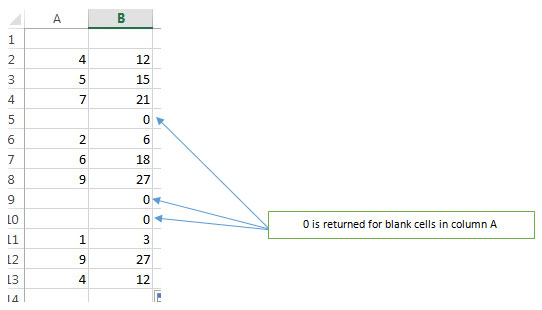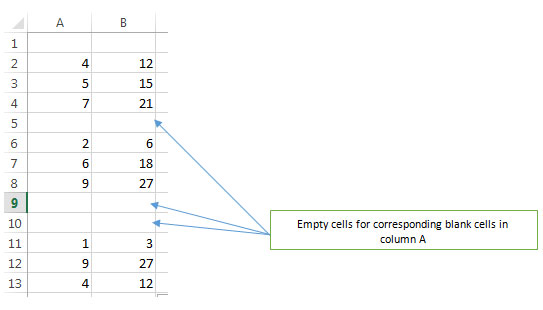# Adjusting a Formula to Return a Blank, Rather than a Zero, When Operating on Empty Cells in Microsoft Excel 2010

In this article we will learn how to adjust a formula to return a blank rather than a zero when operating on empty cells in Microsoft Excel 2010. When you have formulae in a range of cells and these cells include blanks, the formula will return for the empty cells. However you can revise the formulae to show you excel return blank cell instead of 0 whenever there are empty cells in the sheet.

Lets see how to make a cell blank in excel formula.

We have a list in column A which includes numbers as well as blank cells.For example, we have this formula in B2 which multiplies each number in the list by 3 –

=IF(ISBLANK(A2),,A2*3)

• This formula checks if the cell is blank.
• If it is blank, it does nothing, else it multiplies the cell by 3.
• Drag the formula down to the last cell in column B corresponding to the last cell in column A.

The output we get isYou will find that all empty cells in column A are returning 0 in column B. We can avoid this. All we need to do is change the formula from

=IF(ISBLANK(A2),,A2*3)

To

=IF(ISBLANK(A2),””,A2*3)

Note that a pair of double quotes have been included instead of “nothing” so that that formula returns an empty cell when there are 0s in column A.

Edit the formula in B2 and drag it down to the last cell as before.

The output will now be –In this way excel return blank instead of 0 & this makes the data more presentable and easy to read and you can even include headers in between the data elements if needed. You do not have to copy the formula multiple times for each data group which is separated by the blank cells and you can just drag the formula down from the first to the last cell in the range.

1.This helped so much!! Thanks!

2.This works where the user has control over the formulae which use the "blank" values. One instance where it doesn't work is when you are trying to control whether excel draws a line or not between points on an xy chart. If the "y" value is really blank then excel does not draw a line, so your xy values can be the corners of several polygons and excel will "lift the pen up" between polygons. I use this for drawing maps. If the content of the cell is a formula returning an empty string (or even NA) then it is not BLANK, and excel will not lift the pen up. Because of this, a nice map with distinct polygons cannot be produced ib excel with formulas alone.

3.Kilgore - You can use "" instead to account for blanks where there is a formula.

E.g. IF (A2="",,A2*3)

•My issue is that the result shows a date, rather than blank. I've tried all the ideas suggested, but can't get the resulting cell to be blank!
I'm trying to figure out the most recent of two dates, but if there is only one date, to only use the one date listed. This works when either one column or both columns have dates, but returns a "1/1/1900" result where neither columns contain dates.
=IF(ISBLANK(J4:K4),,MAX(J4:K4)) when both columns are empty gives cell # L4 a "1/0/1900" result
=IF(ISBLANK(J5:K5),"",MAX(J5:K5)) - Same result "1/0/1900."
=IF(ISBLANK(J6:K6),"NULL",MAX(J6:K6)) - Same result when both columns are empty: "1/0/1900"

What am I missing, please, to make this work in all three scenarios (1) both columns have a date (2) one or the other column has a date and (3) neither column has a date? Only Scenarios 1 and 2 work thus far

•if you want a blank when both of them are blank then use this formula,
=IF(AND(ISBLANK(J6),ISBLANK(K6))," ",MAX(J6:K6))

Actually ISBLANK works only for one cell reference. when you give a range, it always returns a FALSE.

4.This suggestion only gives the appearance of working. If you follow the above instructions and then put the formula =ISBLANK(B2) in C2 and copy it down through the C column, you will see that B5 is not blank. I am looking for a solution that does not have this problem. I suspect there isn't one because the fact of the matter is that B5 is not blank - it contains a formula.

Terms and Conditions of use

The applications/code on this site are distributed as is and without warranties or liability. In no event shall the owner of the copyrights, or the authors of the applications/code be liable for any loss of profit, any problems or any damage resulting from the use or evaluation of the applications/code.